# GMAT Math : DSQ: Calculating the length of the hypotenuse of an acute / obtuse triangle

## Example Questions

### Example Question #1 : Dsq: Calculating The Length Of The Hypotenuse Of An Acute / Obtuse Triangle

Find the hypotenuse of an obtuse triangle.

Statement 1:  Two given lengths with an inscribed angle.

Statement 2:  Two known angles.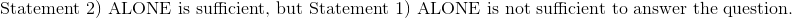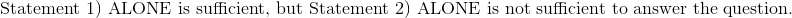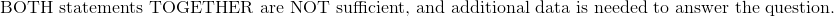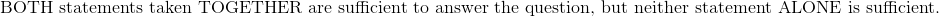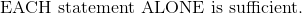Explanation:

Statement 1:  Two given lengths with an inscribed angle.

Draw a picture of the scenario.  The values of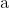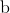, and angle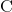are known values.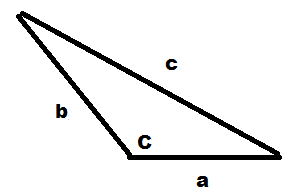Use the Law of Cosines to determine side length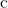.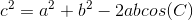Statement 2:  Two known angles.

There is insufficient information to solve for the length of the hypotenuse with only two interior angles.  The third angle can be determined by subtracting the 2 angles from 180 degrees.

The triangle can be enlarged or shrunk to any degree with any scale factor and still yield the same interior angles.  There must also be at least 1 side length in order to calculate the hypotenuse of the triangle by the Law of Cosines.

Therefore:### Example Question #2 : Dsq: Calculating The Length Of The Hypotenuse Of An Acute / Obtuse Triangle

Find the length of the hypotenuse of obtuse triangle TLC:

I)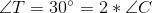II) Side T is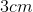Statement II is sufficient to answer the question, but statement I is not sufficient to answer the question.

Both statements are needed to answer the question.

Statement I is sufficient to answer the question, but statement II is not sufficient to answer the question.

Either statement is sufficient to answer the question.

Both statements are needed to answer the question.

Explanation:

Find the length of the hypotenuse of obtuse triangle TLC:

I)II) Side T isUsing I), we can find the measure of all 3 angles: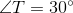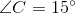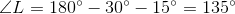Next, use II) and the Law of sines to find the hypotenuse: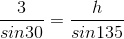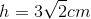And we needed both statements to find it!

### Example Question #3 : Dsq: Calculating The Length Of The Hypotenuse Of An Acute / Obtuse Triangle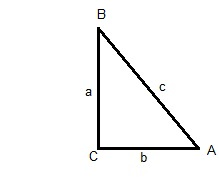For obtuse triangle ABC, what is the length of c?

(1)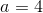and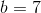(2) c is an integer,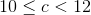Statement (2) ALONE is sufficient, but statement (1) alone is not sufficient.

Statement (1) ALONE is sufficient, but statement (2) alone is not sufficient.

Statements (1) and (2) TOGETHER are NOT sufficient.

BOTH statements (1) and (2) TOGETHER are sufficient to answer the question asked, but NEITHER statement ALONE is sufficient.

EACH statement ALONE is sufficient.

BOTH statements (1) and (2) TOGETHER are sufficient to answer the question asked, but NEITHER statement ALONE is sufficient.

Explanation:

Since this is an obtuse triangle, pythagorean theorem does not apply.

Statement 1 by itself will only determine a range of values c utilizing the 3rd side rule of triangles.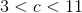.  Therefore, statement 1 alone is insufficient.

Statement 2 by itself will determine that c is either 10 or 11. Therefore, statement 2 alone is insufficient.

When taken together, statements 1 and 2 define a definitive value for c: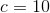. Therefore, BOTH statements (1) and (2) TOGETHER are sufficient to answer the question asked, but NEITHER statement ALONE is sufficient.

### Example Question #4 : Dsq: Calculating The Length Of The Hypotenuse Of An Acute / Obtuse Triangle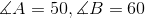.  What is the measure of c?

(1)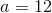(2)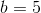BOTH statements TOGETHER are sufficient, but NEITHER statement ALONE is sufficient.

Statement (1) ALONE is sufficient, but statement (2) alone is not sufficient.

Statements (1) and (2) TOGETHER are NOT sufficient.

EACH statement ALONE is sufficient.

Statement (2) ALONE is sufficient, but statement (1) alone is not sufficient.

Statements (1) and (2) TOGETHER are NOT sufficient.

Explanation:

Since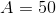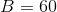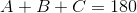, therefore,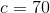thus making this an acute triangle. Pythagorean theorem will not apply.

With the information in statement 1, we can't determine the lengths of any other sides. Therefore, Statement 1 alone is not sufficient.

With the information in statement 2, we can't determine the lengths of any other sides. Therefore, Statement 2 alone is not sufficient.

Using the Third Side Rule for triangles, the information in statements 1 and 2 together would allow us to determine the range of values for c.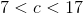, but this does not provide a definitive value for c. Therefore, Both statements together are not sufficient.

Therefore - the correct answer is Statements (1) and (2) TOGETHER are NOT sufficient.

Tired of practice problems?

Try live online GMAT prep today.